# Community

Blog Events Webinars Tutorials Forum
×
Community Blog Similarity Algorithms: Effective Similarity Search in PostgreSQL

# Similarity Algorithms: Effective Similarity Search in PostgreSQL

This post discusses how to calculate the similarity between arrays using algorithms. It focuses on similarity calculation for strings, images, and other types of data in PostgreSQL.

## Background

Similarity analysis is a very common requirement to facilitate search in massive databases. It is employed across multiple business scenarios, including e-commerce websites to search similar products, blog platforms to filter similar blogs, or image hosting services to find similar or duplicate images. In all such cases, on the basis of clues provided by users, a piece of data that is similar to the user's description is filtered out among multiple pieces of text data, image data, and video data.

The similarity between any object or item can be defined in terms of various characteristics of various items, for example, in the case of an online store, a product can be categorized on the basis of size, weight, color, etc. PostgreSQL helps to simplify such search queries based on the similarity of two objects.

This article outlines the traditional similarity calculation approach which is relatively simple and easy to understand. It is quite interesting to know the original technology. First, let's understand how to calculate the similarity between arrays.

## How to Calculate the Similarity between Arrays

Assume that two arrays are available, and each has some elements that are used to represent a user profile. Now, you can calculate the similarity between the arrays of different users to check whether the users have any interests or topics in common.So, next step is how to calculate the similarity between arrays? Several algorithms are used to resolve this challenge.

### Algorithm Introduction

To begin with, the similarity analysis, let's have a quick look at the basic elements of similarity calculation. The following describes several terms about the array:

• Na, Nb – the number of unique elements in the arrays
• Nu – the number of unique elements in the union of sets
• Ni – the number of unique elements in the intersection of arrays

Now, moving ahead, let's understand various algorithms:

1. The simplest similarity algorithm is as follows: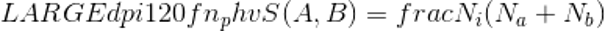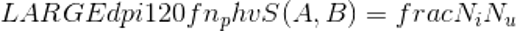This algorithm has the following benefits:

• It is easy to understand
• Speed of calculation: N × log (N)
• Provides sound support even when Nb and Na are large
1. Another similarity algorithm is as follows: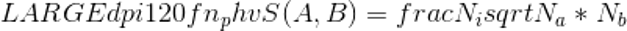The benefits of this algorithm are as follows:

• Speed of calculation: N × log (N)
• Provides sound support even when Nb and Na are large

However, it is important to note that both of these methods face similar challenges, such as.

• Few Elements: Both methods result in large scatter of similarity. In other words, when the number of elements is small, the similarity is greatly scattered.
• Frequent Elements: When an element frequently appears, the required TF weight is not available and thus the reasonable similarity cannot be obtained in both the methods.
1. The TF-IDF coefficient solves the preceding problems.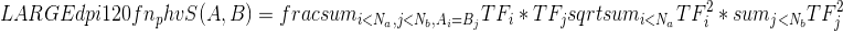Wherein,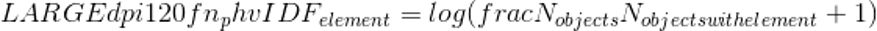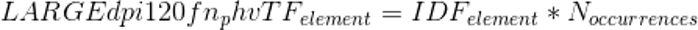Now, since we have the theoretical knowledge, let's perform the similarity calculation.

PostgreSQL is easy to scale and therefore, you don't need to heavily modify the kernel configuration of PostgreSQL. Just add a plug-in called, smlar plug-in and you are good to go. smlar plug-in supports similarity formulas and allows to customize similarity algorithm formulas. Besides, the smlar plug-in supports the GiST and GIN indexes.

### The smlar Plug-in for Similarity Calculation

Follow the steps below to get started with similarity calculation using the smalar pugin:

Step 1. Deploy the smlar plug-in using the following commands.

``````git clone git://sigaev.ru/smlar
cd smlar
USE_PGXS=1 make
USE_PGXS=1 make install
``````

Step 2. Set the parameter and similarity threshold. When the similarity value is greater than the threshold, TRUE is returned; otherwise, FALSE is returned.

``````smlar.threshold = 0.8  # or any other value >0 and <1
``````

Step 3. Now, let's test the installed smlar plug-in.

``````psql

test=# CREATE EXTENSION smlar;
CREATE EXTENSION
``````

Step 4. Calculate the similarity as shown below.

``````test=# SELECT smlar('{1,4,6}'::int[], '{5,4,6}' );
smlar
----------
0.666667
(1 row)

test=# SELECT smlar('{1,4,6}'::int[], '{5,4,6}', 'N.i / sqrt(N.a * N.b)' );
smlar
----------
0.666667
(1 row)
``````

Step 5. Determine whether the two arrays are similar, based on the similarity threshold.

``````test=# SELECT '{1,4,6,5,7,9}'::int[] % '{1,5,4,6,7,8,9}'::int[] as similar;
similar
---------
t
(1 row)
``````

Index support. The % operator supports index retrieval to quickly obtain the data that you want to query.

GiST/GIN support for % operation. The parameter "similar.type" allows you to specify the kind of formula that can be used to calculate the similarity, including cosine (default), overlap or tfidf. For "tfidf", you need to make additional configuration. For this article, we will not cover this topic in detail, you can refer to README file for all the details.

Now, let's consider an example to understand the use of this extension. As mentioned above, three formulas are available for similarity algorithms, and all three are applicable here. You can also customize formulas to calculate the similarity. You can provide a specific formula for calculating the similarity.

``````test=# SELECT smlar('{1,4,6}'::int[], '{5,4,6}', 'N.i / sqrt(N.a * N.b)' );
smlar
----------
0.666667
(1 row)
``````

## Similarity Calculation for Strings, Images, and Other Types of Data

Methods for calculating the similarity between arrays have been described above. The following sections describe how to calculate the similarity between strings, images, and other types of data.

### String Similarity

You can also calculate the similarity between strings using different methods. For example, the PostgreSQL pg_trgm plug-in divides strings into many tokens and then calculates the similarity based on the tokens. The following command shows the calculation of the similarity between arrays.

``````postgres=# select similarity('hello digoal','hell digoal');
similarity
------------
0.785714
(1 row)
``````

The pg_trgm plug-in is easy to use and supports many types of index retrieval and sorting, including the indexing of regular expressions.

### Image Similarity

This section describes how to calculate the similarity between images. Moreover, images can be digitized as shown in the following example, where a large image is compressed into a smaller image: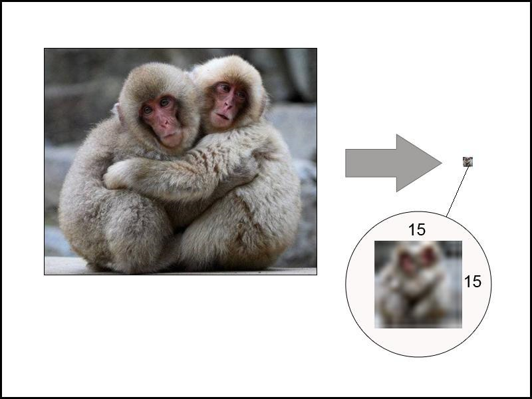For instance, here the large image is compressed into a smaller image of 15 x 15 pixels. The image size of 15 x 15 pixels contains 225 cells in total. Each cell consists of three primary colors, which are red, green, and blue (RGB). You can calculate the RGB of each cell into value and eventually obtain a matrix array with 15 × 15 cells.

For example, the value of a cell is 0.299 × red + 0.587 × green + 0.114 × blue. Again, it leads to the calculation of similarity between arrays.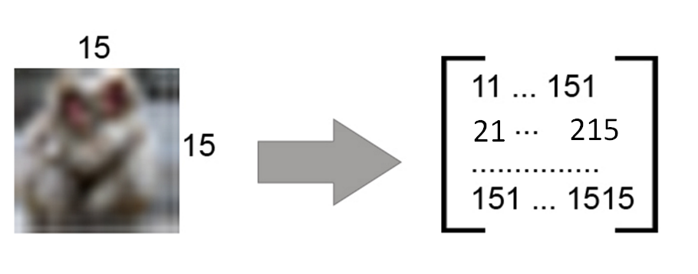The following example uses the preceding method to calculate the similarity between images.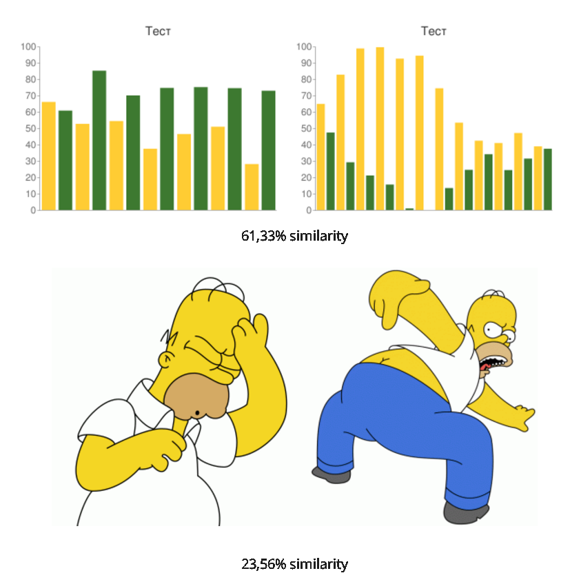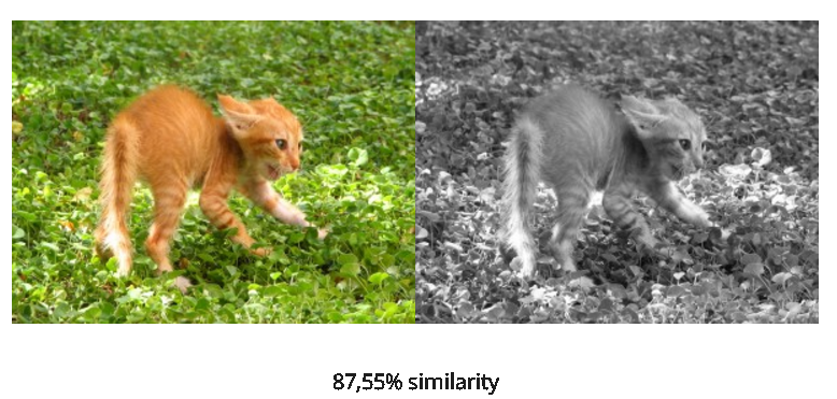Isn't it amazing? Now, let's consider an example to show how to use PostgreSQL for searching.

``````CREATE TABLE images (
id serial PRIMARY KEY,
name varchar(50),
image_array integer[]
);

INSERT into images(image_array) VALUES ('{1010257,...,2424257}');

test=# SELECT count(*) from images;
count
--------
200000
(1 row)

test=# EXPLAIN ANALYZE SELECT id FROM images WHERE images.image_array % '{1010259,...,2424252}'::int[];

Aggregate  (cost=14.58..14.59 rows=1 width=0) (actual time=1.785..1.785 rows=1 loops=1)
-&gt;  Seq Scan on images  (cost=0.00..14.50 rows=33 width=0) (actual time=0.115..1.772 rows=20 loops=1)
Filter: (image_array % '{1010259,1011253,...,2423253,2424252}'::integer[])
Total runtime: 5152.819 ms
(4 rows)

CREATE INDEX image_array_gin ON images USING GIN(image_array _int4_sml_ops);

or

CREATE INDEX image_array_gist ON images USING GIST(image_array _int4_sml_ops);
``````

Now, use test and check the performance

``````test=# EXPLAIN ANALYZE SELECT id FROM images WHERE images.image_array % '{1010259,1011253,...,2423253,2424252}'::int[];

Aggregate  (cost=815.75..815.76 rows=1 width=0) (actual time=320.428..320.428 rows=1 loops=1)
-&gt;  Bitmap Heap Scan on images  (cost=66.42..815.25 rows=200 width=0) (actual time=108.127..304.524 rows=40000 loops=1)
Recheck Cond: (image_array % '{1010259,1011253,...,2424252}'::integer[])
-&gt;  Bitmap Index Scan on image_array_gist  (cost=0.00..66.37 rows=200 width=0) (actual time=90.814..90.814 rows=40000 loops=1)
Index Cond: (image_array % '{1010259,1011253,...,2424252}'::integer[])
Total runtime: 320.487 ms
(6 rows)

test=# SELECT count(*) from images;
count
---------
1000000
(1 row)

test=# EXPLAIN ANALYZE SELECT count(*) FROM images WHERE images.image_array % '{1010259,1011253,...,2423253,2424252}'::int[];

Bitmap Heap Scan on images  (cost=286.64..3969.45 rows=986 width=4) (actual time=504.312..2047.533 rows=200000 loops=1)
Recheck Cond: (image_array % '{1010259,1011253,...,2423253,2424252}'::integer[])
-&gt;  Bitmap Index Scan on image_array_gist  (cost=0.00..286.39 rows=986 width=0) (actual time=446.109..446.109 rows=200000 loops=1)
Index Cond: (image_array % '{1010259,1011253,...,2423253,2424252}'::integer[])
Total runtime: 2152.411 ms
(5 rows)

EXPLAIN ANALYZE SELECT smlar(images.image_array, '{1010259,...,2424252}'::int[]) as similarity FROM images WHERE images.image_array % '{1010259,1011253, ...,2423253,2424252}'::int[] ORDER BY similarity DESC;

Sort  (cost=4020.94..4023.41 rows=986 width=924) (actual time=2888.472..2901.977 rows=200000 loops=1)
Sort Key: (smlar(image_array, '{...,2424252}'::integer[]))
Sort Method: quicksort  Memory: 15520kB
-&gt;  Bitmap Heap Scan on images  (cost=286.64..3971.91 rows=986 width=924) (actual time=474.436..2729.638 rows=200000 loops=1)
Recheck Cond: (image_array % '{...,2424252}'::integer[])
-&gt;  Bitmap Index Scan on image_array_gist  (cost=0.00..286.39 rows=986 width=0) (actual time=421.140..421.140 rows=200000 loops=1)
Index Cond: (image_array % '{...,2424252}'::integer[])
Total runtime: 2912.207 ms
(8 rows)
``````

## Text Similarity Analysis

Text analysis refers to converting text to ts_vector data by using full-text retrieval and then performing similarity analysis for full-text search (FTS). We will not be covering this topic in this article.

## Better Methods for Image Similarity Analysis

There are better technologies available for image search which are more reasonable than the preceding pixel matrix calculation methods. For example, the Haar wavelet algorithm, that is is integrated into PostgreSQL.

``````float4 smlar(anyarray, anyarray)
- computes similary of two arrays. Arrays should be the same type.

float4 smlar(anyarray, anyarray, bool useIntersect)
-  computes similary of two arrays of composite types. Composite type looks like:
CREATE TYPE type_name AS (element_name anytype, weight_name FLOAT4);
useIntersect option points to use only intersected elements in denominator
see an exmaples in sql/composite_int4.sql or sql/composite_text.sql

float4 smlar( anyarray a, anyarray b, text formula );
- computes similary of two arrays by given formula, arrays should
be the same type.
Predefined variables in formula:
N.i   - number of common elements in both array (intersection)
N.a   - number of uniqueelements in first array
N.b   - number of uniqueelements in second array
Example:
smlar('{1,4,6}'::int[], '{5,4,6}' )
smlar('{1,4,6}'::int[], '{5,4,6}', 'N.i / sqrt(N.a * N.b)' )
That calls are equivalent.

anyarray % anyarray
- returns true if similarity of that arrays is greater than limit

float4 show_smlar_limit()  - deprecated
- shows the limit for % operation

float4 set_smlar_limit(float4) - deprecated
- sets the limit for % operation

Use instead of show_smlar_limit/set_smlar_limit GUC variable
smlar.threshold (see below)

text[] tsvector2textarray(tsvector)
- transforms tsvector type to text array

anyarray array_unique(anyarray)
- sort and unique array

float4 inarray(anyarray, anyelement)
- returns zero if second argument does not present in a first one
and 1.0 in opposite case

float4 inarray(anyarray, anyelement, float4, float4)
- returns fourth argument if second argument does not present in
a first one and third argument in opposite case

GUC configuration variables:

smlar.threshold  FLOAT
Array's with similarity lower than threshold are not similar
by % operation

smlar.persistent_cache BOOL
Cache of global stat is stored in transaction-independent memory

smlar.type  STRING
Type of similarity formula: cosine(default), tfidf, overlap

smlar.stattable STRING
Name of table stored set-wide statistic. Table should be
defined as
CREATE TABLE table_name (
value   data_type UNIQUE,
ndoc    int4 (or bigint)  NOT NULL CHECK (ndoc>0)
);
And row with null value means total number of documents.
See an examples in sql/*g.sql files
Note: used on for smlar.type = 'tfidf'

smlar.tf_method STRING
Calculation method for term frequency. Values:
"n"     - simple counting of entries (default)
"log"   - 1 + log(n)
"const" - TF is equal to 1
Note: used on for smlar.type = 'tfidf'

smlar.idf_plus_one BOOL
If false (default), calculate idf as log(d/df),
if true - as log(1+d/df)
Note: used on for smlar.type = 'tfidf'

Module provides several GUC variables smlar.threshold, it's highly
custom_variable_classes = 'smlar'       # list of custom variable class names
smlar.threshold = 0.6  #or any other value > 0 and < 1
and other smlar.* variables

GiST/GIN support for % and  && operations for:
Array Type   |  GIN operator class  | GiST operator class
---------------+----------------------+----------------------
bit[]         | _bit_sml_ops         |
bytea[]       | _bytea_sml_ops       | _bytea_sml_ops
char[]        | _char_sml_ops        | _char_sml_ops
cidr[]        | _cidr_sml_ops        | _cidr_sml_ops
date[]        | _date_sml_ops        | _date_sml_ops
float4[]      | _float4_sml_ops      | _float4_sml_ops
float8[]      | _float8_sml_ops      | _float8_sml_ops
inet[]        | _inet_sml_ops        | _inet_sml_ops
int2[]        | _int2_sml_ops        | _int2_sml_ops
int4[]        | _int4_sml_ops        | _int4_sml_ops
int8[]        | _int8_sml_ops        | _int8_sml_ops
interval[]    | _interval_sml_ops    | _interval_sml_ops
money[]       | _money_sml_ops       |
numeric[]     | _numeric_sml_ops     | _numeric_sml_ops
oid[]         | _oid_sml_ops         | _oid_sml_ops
text[]        | _text_sml_ops        | _text_sml_ops
time[]        | _time_sml_ops        | _time_sml_ops
timestamp[]   | _timestamp_sml_ops   | _timestamp_sml_ops
timestamptz[] | _timestamptz_sml_ops | _timestamptz_sml_ops
timetz[]      | _timetz_sml_ops      | _timetz_sml_ops
varbit[]      | _varbit_sml_ops      |
varchar[]     | _varchar_sml_ops     | _varchar_sml_ops
``````

## References

Build your own PostgreSQL solution on Alibaba Cloud with ApsaraDB for RDS PostgreSQL.

0 0 0
Share on

# Related Products

• ##Database Overview

Fully managed and less trouble database services

• ##AnalyticDB for PostgreSQL

An online MPP warehousing service based on the Greenplum Database open source program

• ##Image Search

An intelligent image search service with product search and generic search features to help users resolve image search requests.

• ##OpenSearch

OpenSearch helps develop intelligent search services.